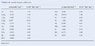# P-Chem: Van der Waals Equation data

bubbaburp

## Homework Statement

Based on values for the Van der Waals equation tabulated in Table 1.6 in
appendix A, determine an approximate molecular volume for CCl4 and CH4.

Rationalize these values based on reasonable bond lengths and other
parameters. The Lennard-Jones potential energy function is often used to
model interactions between molecules.

## Homework Equations

V(r) = 4ε [{σ/R}^12 - {σ/R}^6]

For Cl, ε = 357/k, σ = 4.115 Angstrom
For H, ε = 33.3/k, σ = 2.968 Angstrom

## The Attempt at a Solution

No attempt has been made as of yet, except for logging on this site and requiring assistance!

#### Attachments

•van der Waals coefficients.jpg
22.5 KB · Views: 405
Last edited: